Quick Notes Mass Spectrometry - Time of Flight (ToF)

• Time of Flight Mass spectrometry is an analytical technique that is used to find the relative mass of an element or molecule.
• There are four stages in a time of flight mass spectrometer: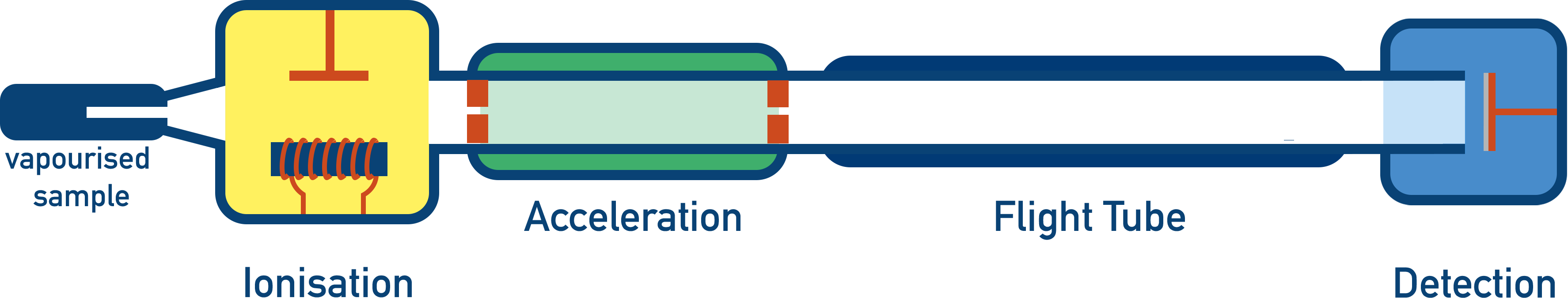• Ionisation - Sample is given a positive charge and becomes a positively charged ion. There are two possible methods: electron impact and electrospray ionisation.
• AccelerationPositively charged ions are accelerated to ensure they all have the same kinetic energy.
• Flight TubeCharged ions have to travel a certain distance in the flight tube. Heavier ions will take longer to travel through the flight tube compared to lighter ions.
• DetectionIons hit a negatively charged detector plate and a signal is generated. The greater the signal, the greater the number of ions hitting the plate at that moment.
• The velocity of the ion is found by using the time taken for the ion to travel a certain distance in the flight tube.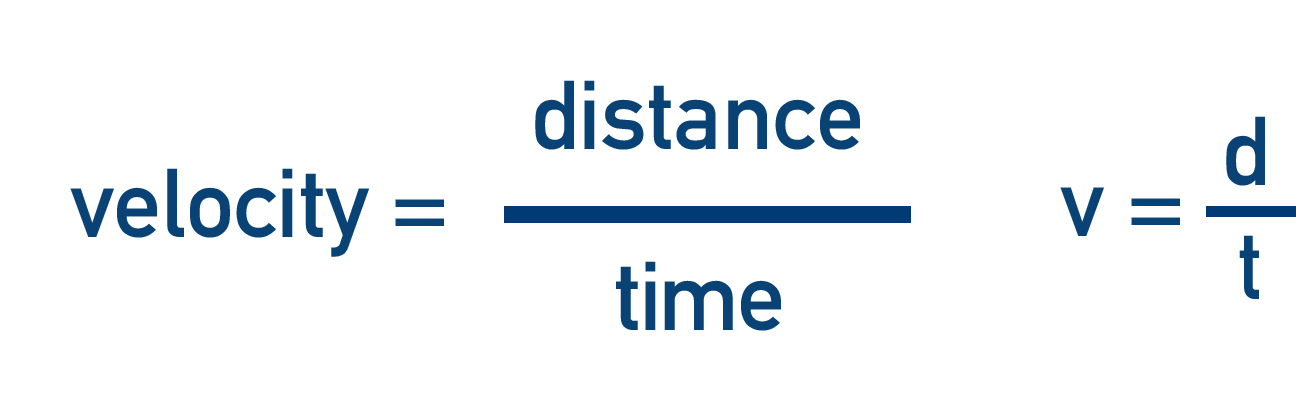• The velocity is then used to find the mass of the ion.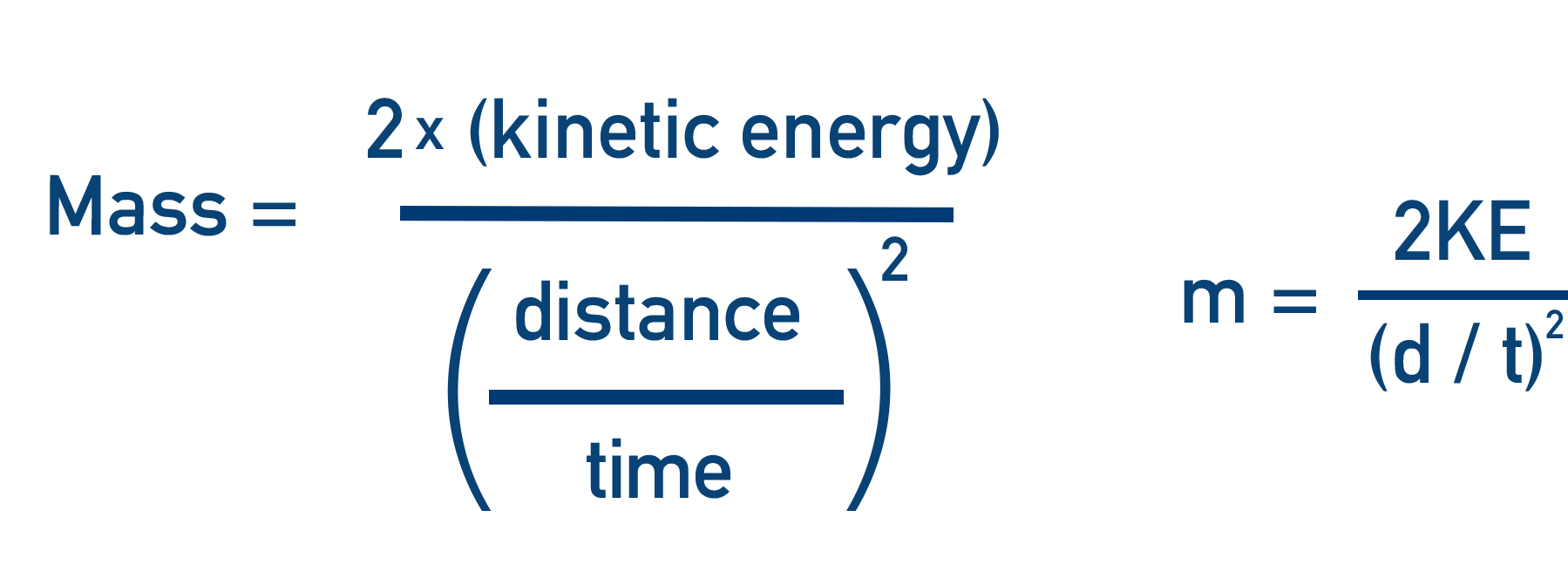Full Notes Mass Spectrometry - Time of Flight (ToF)

NOTE - Mass spectrometry is an analytical technique that is used to find the relative mass of an element or molecule. The machine used in mass spectrometry is called a mass spectrometer, and ‘time of flight mass spectrometry’ refers to one particular type of mass spectrometer. Unless your exam board specifically mentions time of flight mass spectrometry, you probably won’t need to know this page and only need to understand the general theory behind mass spectrometry - see Mass Spectrometry.

A time of flight mass spectrometer works on the principle that, if given the same amount of energy, heavier particles will travel with a slower speed than lighter particles. In the spectrometer, particles are made to move through a long tube and the time it takes the particles to move from one end to the other is used to find their velocities (speed*). The velocity of a particle, with the use of some simple equations, can be used to find its (relative) mass.

There are four key stages to a time of flight mass spectrometer:1. Ionisation

2. Acceleration

3. Flight Tube ('Time of Flight')

4. Detection

## 1. Ionisation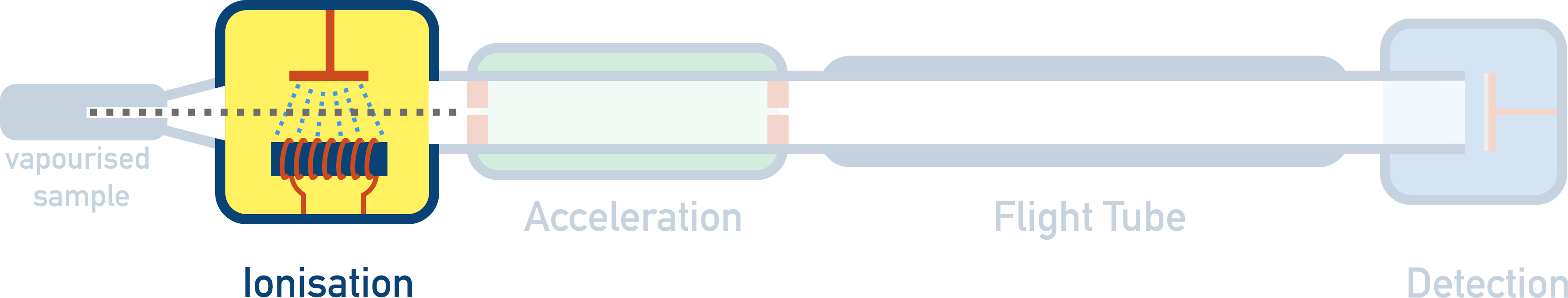Sample is given a positive charge and becomes a positively charged ion.

In order for mass spectrometry to work, we need to be able to force a sample to accelerate in a particular direction. To enable this to happen, the sample is given a positive charge in a process called ‘ionisation’. Once the particle has a positive charge, it can be accelerated with the use of electrical fields and charged plates. There are two ways to ionise a sample in a time of flight mass spectrometer - electron impact and electrospray ionisation.

Electron impact (also known as electron ionisation) is a simple method of ionisation where a sample is vaporised (turned into gas) and a beam of high energy electrons is fired at the sample. An electron is ‘knocked’ out of its orbit around an atom or molecule, forming a 1+ ion.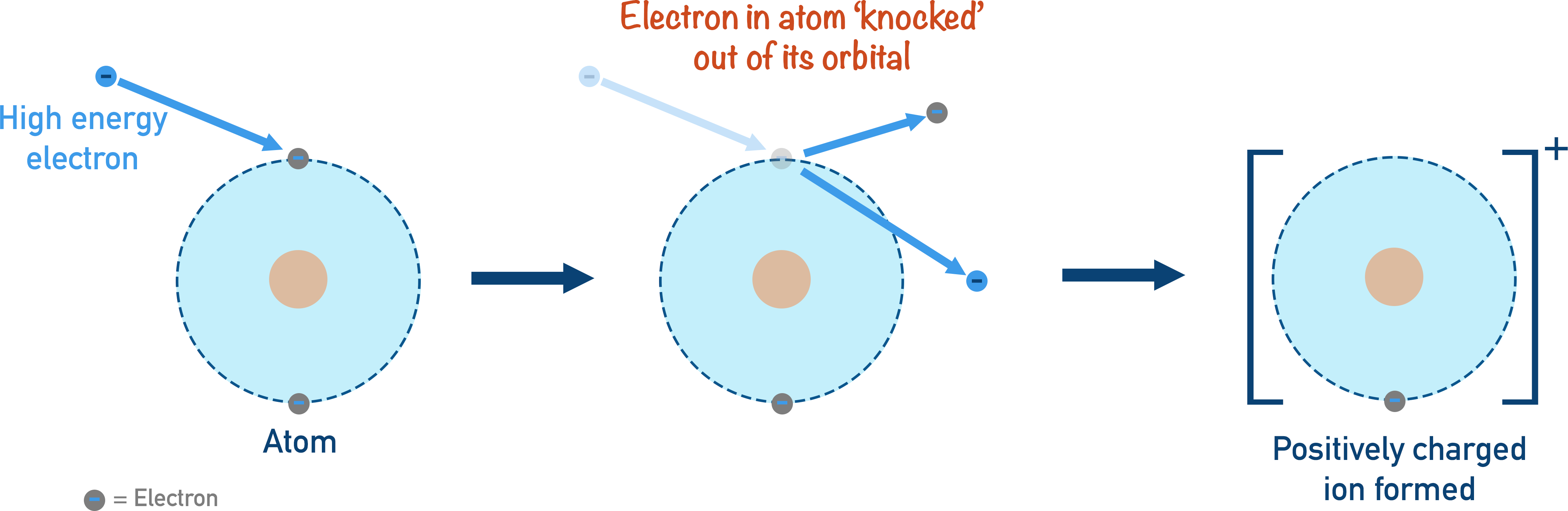When larger molecules are ionised, the resulting positively charged molecular ion is often highly unstable and will ‘break up’ into smaller pieces called fragments. The detection of these fragments can give useful information about the possible structure of the molecule being analysed. Electron impact ionisation is a good method for analysing the masses of elements and samples with low formula masses. Very large molecules simply get broken apart during ionisation and for those, electrospray ionisation is used.

Electrospray ionisation is where a proton (positively charged H+ ion) is added to the sample to give it a positive charge. Unlike electron impact ionisation (see above), no fragmentation occurs and the sample remains complete.

During electrospray ionisation, a sample is dissolved in a volatile solvent and injected into the mass spectrometer through a hollow, metal needle. The needle is given a positive charge (by connecting it to a positive electrical terminal), and as the sample is forced through the charged needle it gains a positively charged proton (H+) and a fine mist of positively charged ions is produced.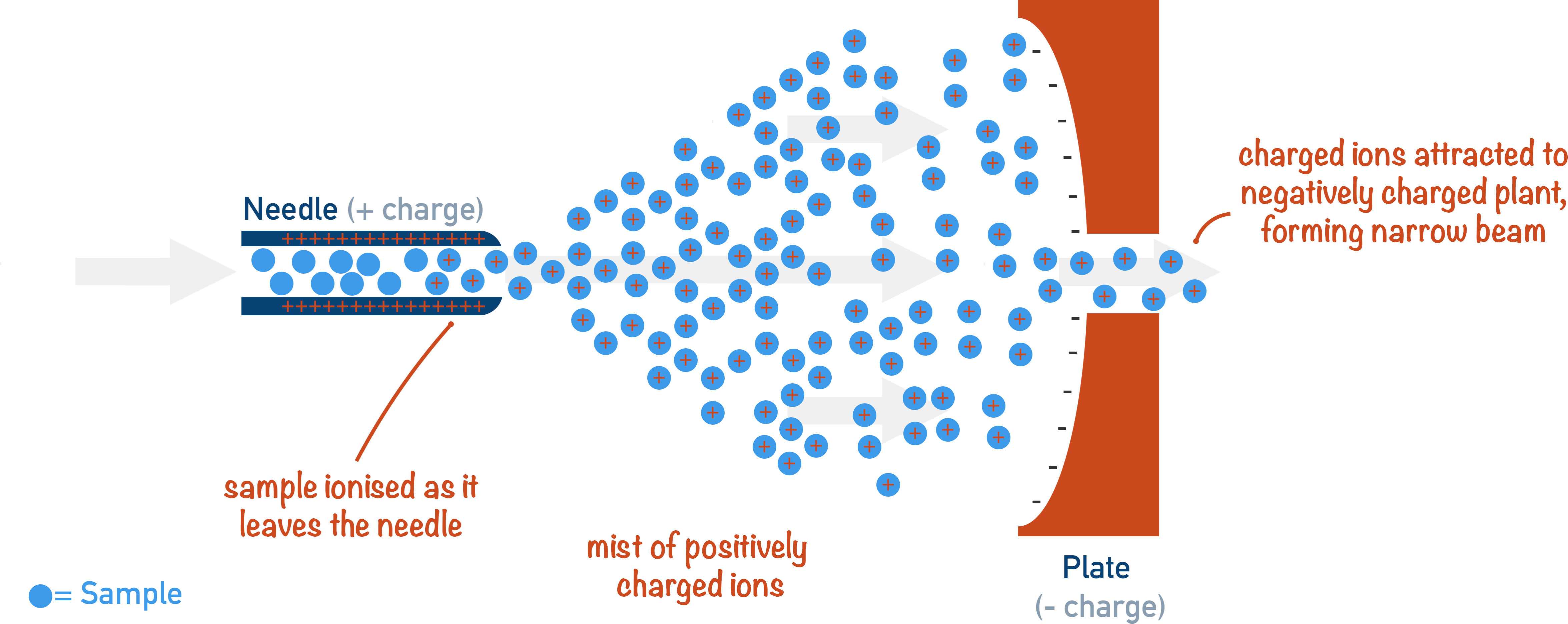M(g) + H+ → MH+(g)

The volatile solvent in the mist vaporises (due to its high volatility) and the positively charged sample ions are ready to be accelerated. The sample rarely breaks apart into fragments as no electron has been removed (unlike in electron impact ionisation), making it very useful for large molecules (such as proteins).

## 2. Acceleration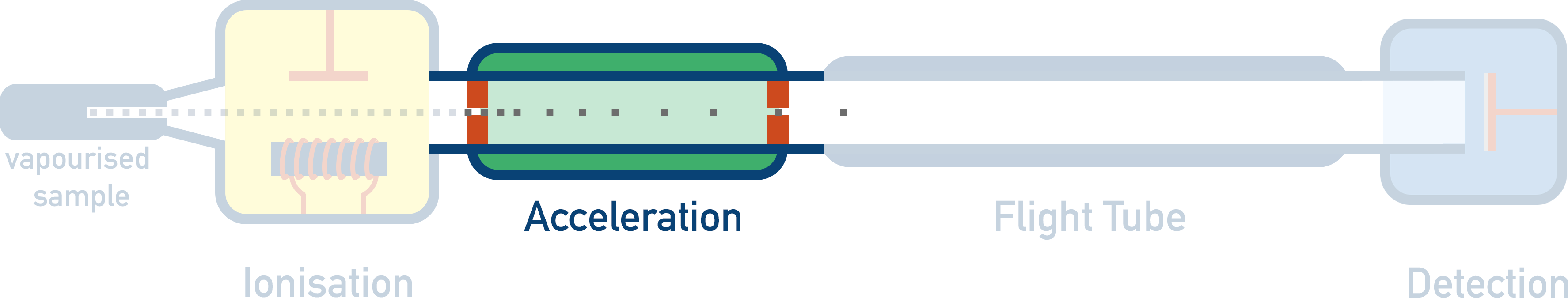Positively charged ions are accelerated to ensure they all have the same kinetic energy.

After the sample has been ionised, the positively charged ions are accelerated by an electrical field. As opposite charges attract, the positively charged ions are made to ‘rush’ towards negatively charged plates, accelerating as they move.By accelerating the sample ions, we are making sure that they all have the same kinetic energy. This is important as it means that any difference in velocity between ions in the flight tube is based only on their masses (or ‘mass to charge ratio’) - see the equation below.

If given the same amount of kinetic energy, heavier ions will have a slower velocity and lighter ions will have a faster velocity.

## 3. Flight Tube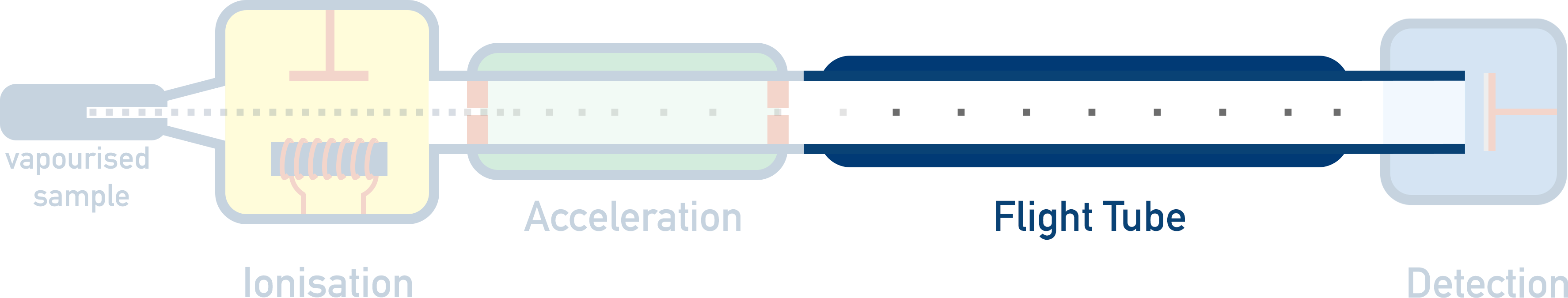Time taken for ions to move though a flight tube is measured. The time taken is based on the ion’s mass and is used to find the velocity of the particle(s).

Once the ions have been accelerated, they are ready to be analysed!

The positively charged ions are forced to go through a small tube (called a ‘flight tube’). As all the particles have the same amount of kinetic energy, the time it takes for an ion to move through this tube is directly related to the mass of the particle - heavier ions will take longer to travel the same distance as lighter ions. If the distance of the flight tube and the time it takes the ion to move through it are known, the velocity can be calculated.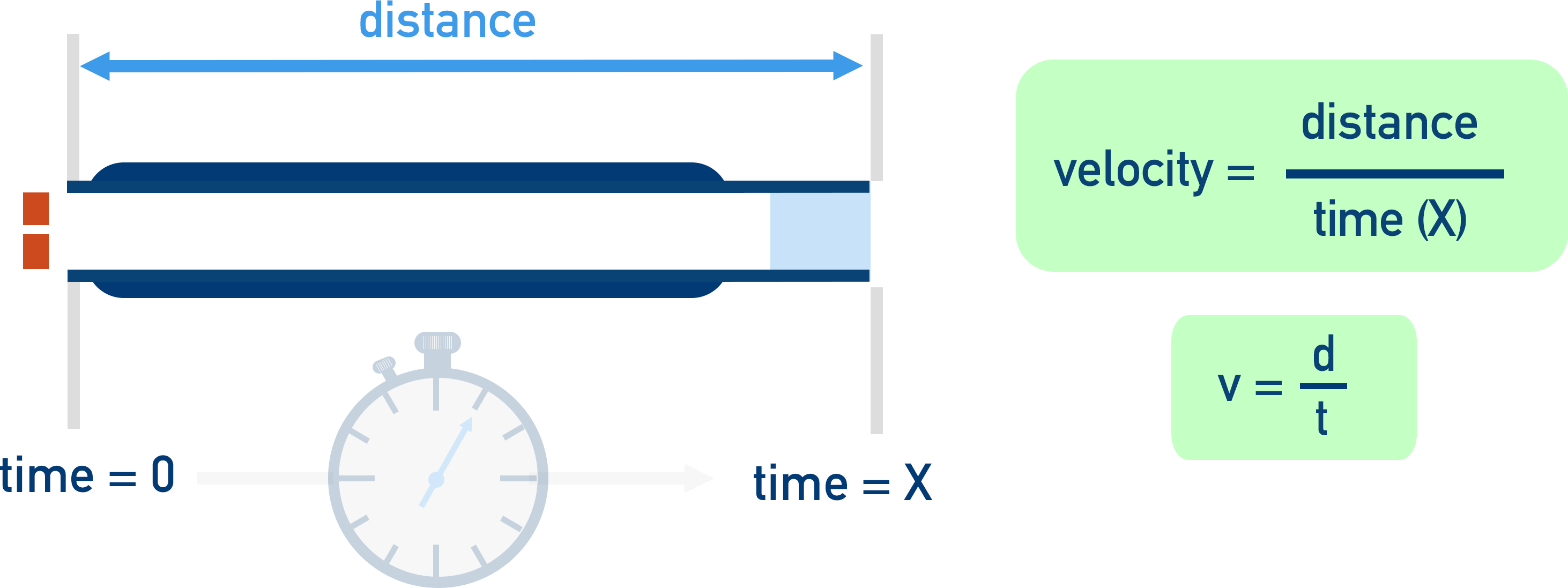v = d / t

Combining the equation for kinetic energy and velocity, we get:

t = d √(m / 2KE)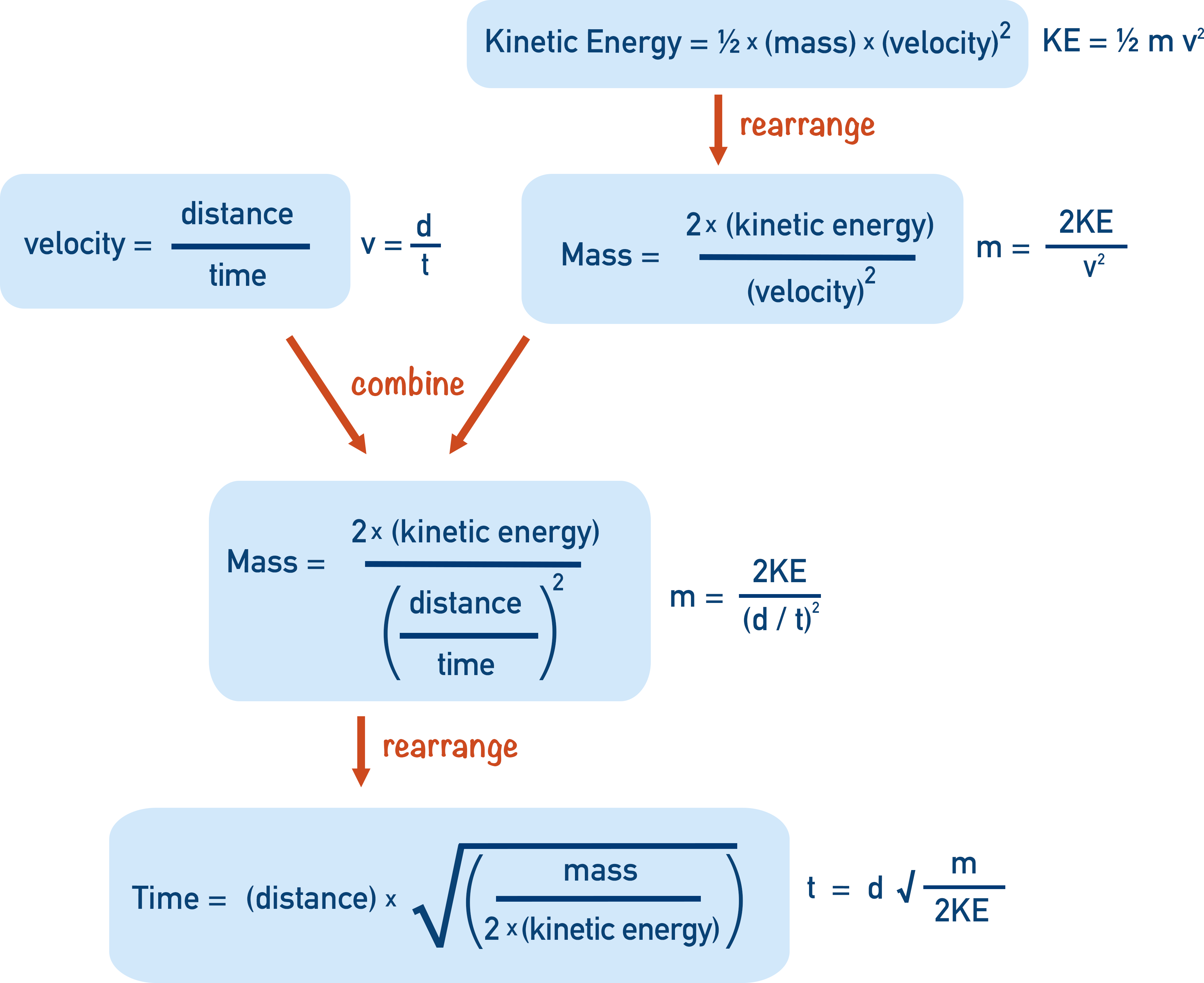If a sample of an element containing several isotopes is accelerated and enters the flight tube, the lighter isotope ions will travel through the flight tube faster than the heavier ions.

## 4. Detection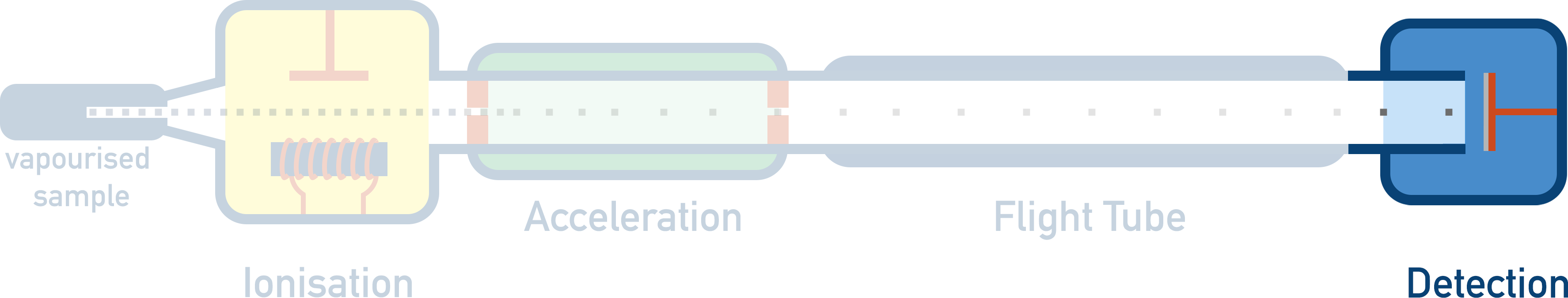Ions hit a negatively charged detector plate and a signal is generated. The greater the signal at a particular time, the greater the number of ions hitting the plate.

Light particles are very easily blown; they take a very small force of air (magnetic field!) to move around the corner perfectly.

When the ions have reached the end of the flight tube, they hit a detector plate that is negatively charged with electrons on its surface.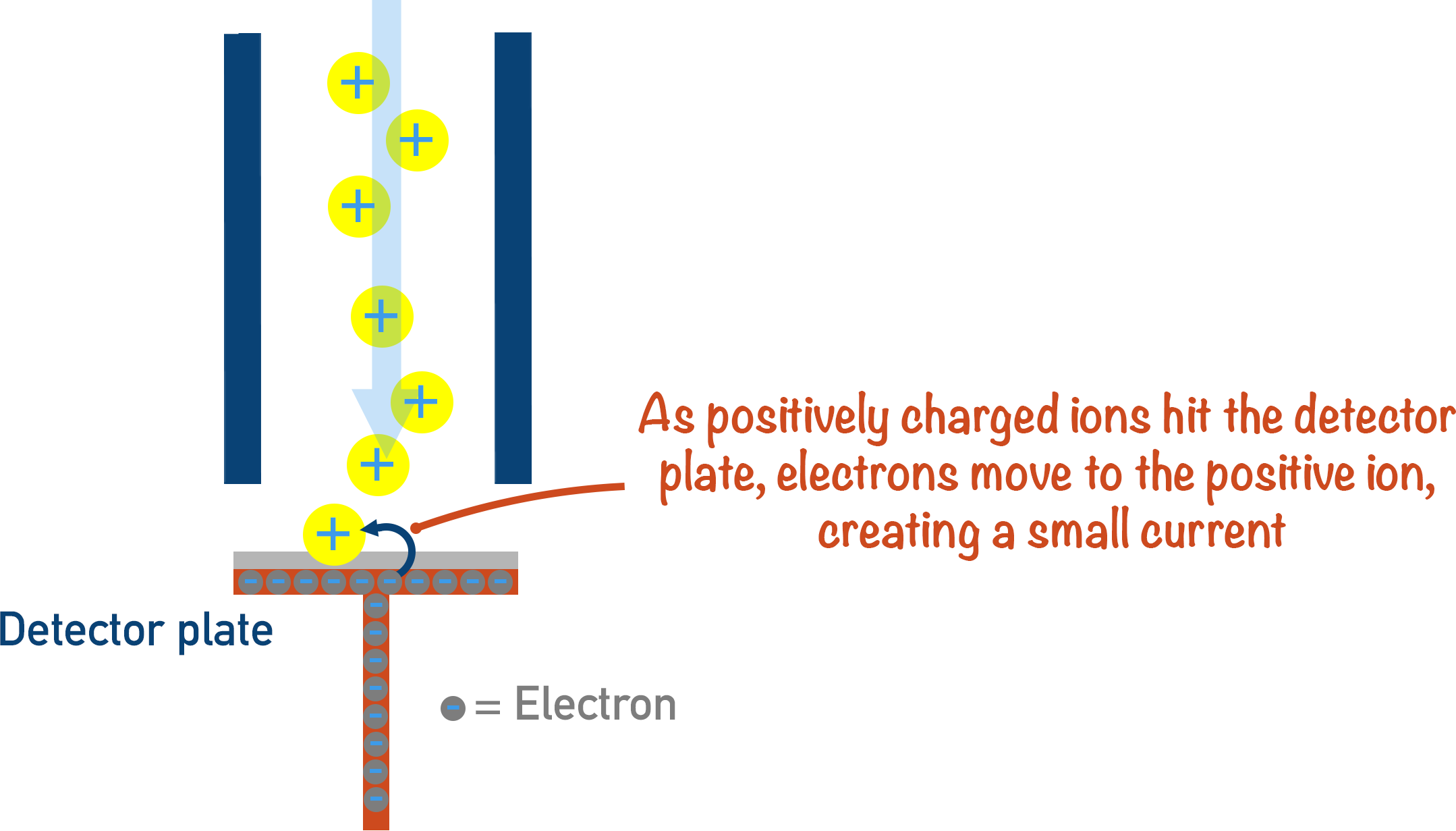When a positively charged ion hits the detector plate, an electron leaves the surface of the plate and joins the ion - making it neutral. Each time this happens, a very small electrical current is produced and this is recorded. The size of the current recorded is based on the number of particles hitting the plate. The more ions hitting the plate at any one moment in time, the greater the signal.

A computer organises the signals received from the detector and produces a mass spectra.

## Using the Data Produced

There are two bits of data that can be obtained from a time of flight mass spectrometer - the time it takes a particular ion to move through the flight tube and the amount of those ions in the sample compared to other ions (based on the strengths of all signals compared to each other).

The time taken for an ion to move through the flight tube can be used to find its relative mass (or mass to charge ratio); the amounts of different ions can be compared to each other to show the ‘abundance’ of the particular ion. From both the relative masses of all ions in the sample and their abundances, the relative atomic mass of an element can be determined.## M+1 Peak

If a molecule of a substance contains 2H or 13C (naturally occurring heavier isotopes of carbon and hydrogen), the molecule will have a relative mass that is one heavier than other molecules of the same substance. This is seen on mass spectra as a ‘M + 1’ peak.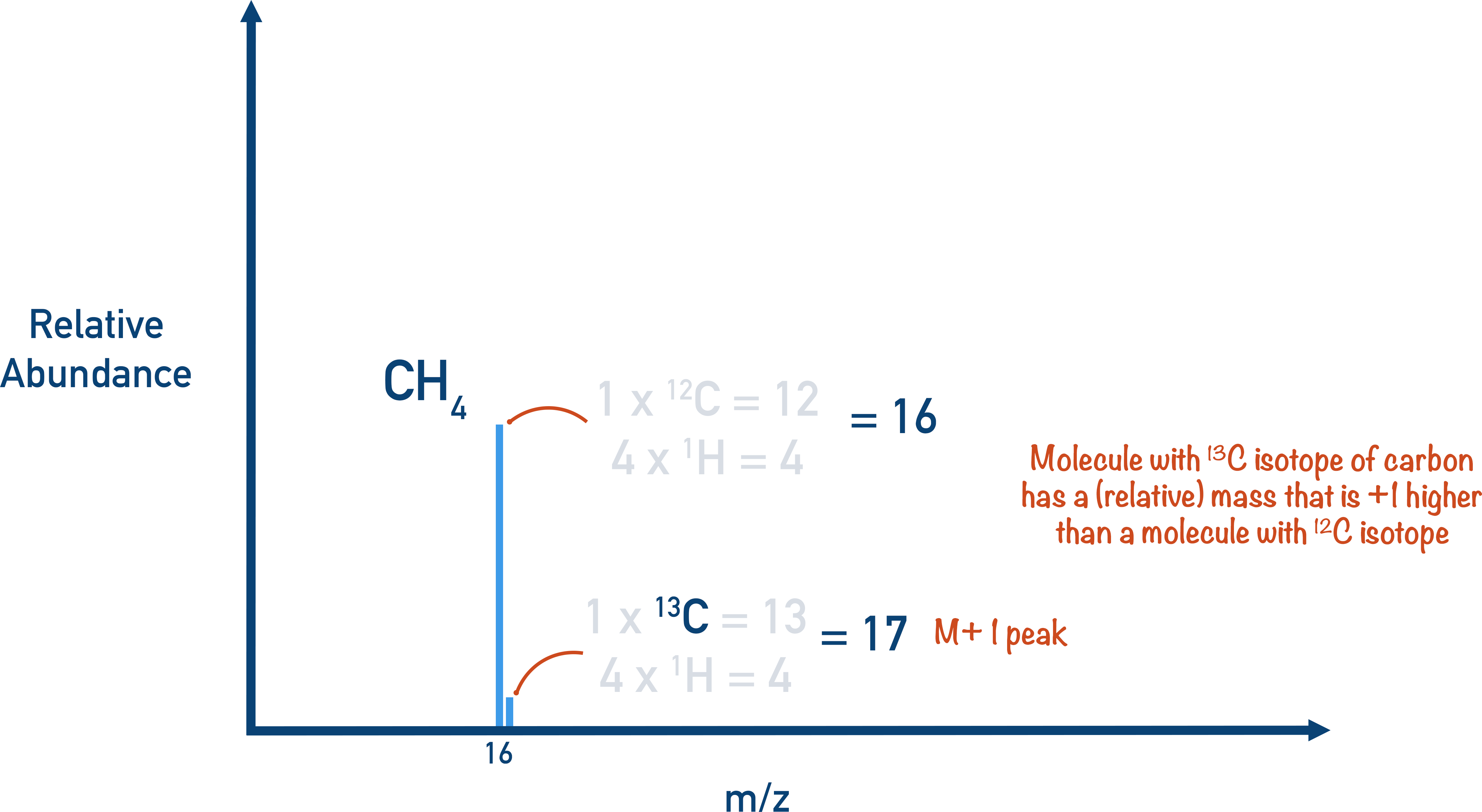The M + 1 peak is always much smaller than the main molecular ion peak as the natural abundance of 2H and 13C is low, meaning most molecules won’t contain any. On some mass spectra, the M + 1 peak is removed.

*From the top of the page - Technically, it is the velocity of the particles that we need to consider in mass spectrometry, rather than simply their ‘speed’. The reasons for this are completely mechanical and based in physics - speed is a scalar quantity and velocity is a vector quantity. The vector quantity of velocity can be linked to the kinetic energy and acceleration of a moving object, this is why velocity is referred to. None of this is relevant for A-level Chemistry students – all you need to remember is to refer to velocity rather than speed.